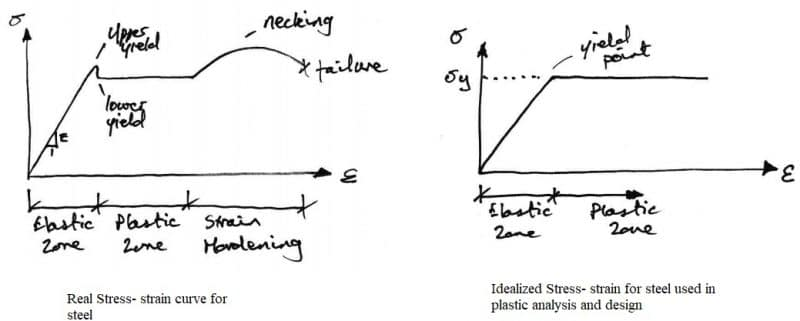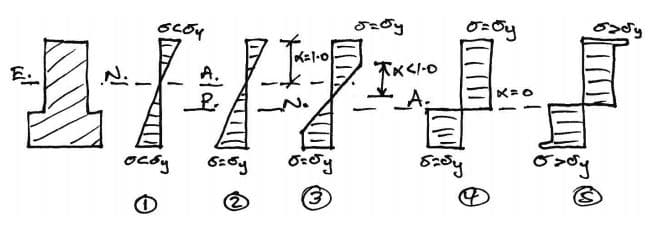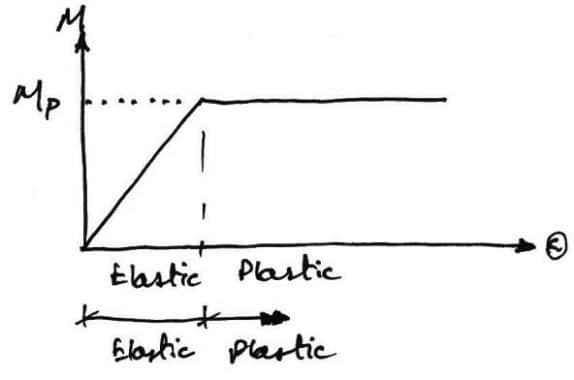# Bases of Plastic Analysis of Beams and Portal Frames

Plastic analysis is the method through which the actual failure load of a structure is calculated. This failure load can be significantly greater than the elastic load capacity.

In plastic analysis and design of a structure, the ultimate load of the structure as a whole is regarded as the design criterion. The term plastic has occurred due to the fact that the ultimate load is found from the strength of steel in the plastic range.

This method is rapid and provides a rational approach for the analysis of the structure. Plastic analysis and design have its main application in the analysis and design of statically indeterminate frames.

Plastic analysis is based on the idealization of the stress-strain curve as elastic-perfectly-plastic. It is further assumed that the width-thickness ratio of plate elements is small so that local buckling does not occur- in other words, the sections are classified as plastic.

## What are the Bases of Plastic Analysis of Beams and Frames?

### 1. Material Behavior

The actual behavior of ductile materials such as mild steel which is represented through the stress-strain curve is shown in Fig.1. it is noted that the material can sustain strains far in excess of the strain at which yield occurs before failure. This property of the material is called its ductility.

However, an idealized stress-strain curve, which is shown in Fig. 1, is assumed and used for plastic design. It can be seen that, once the yield has been reached it is taken that an indefinite amount of strain can occur.

Due to the fact that so much post-yield strain is assumed, the actual material must be capable of allowing such strains i.e. it should be adequately ductile for the idealized stress-strain curve to be valid.Fig. 1: Real and Idealized Stress- strain of Steel

### 2. Cross-Section Behavior

#### 2.1 Moment-Rotation Characteristics of General Cross Section

The moment-rotation characteristics of a general cross-section are described using an arbitrary cross-section with a vertical plane of symmetry. As illustrated in Fig. 2, it is assumed that the cross-section subjected to an increasing bending moment, and variations of stress in this cross-section is presented below.Fig. 2: Distribution of Stress in the Cross- section of Steel

Elastic Behavior

At stage 1, the applied moment causes stresses over the cross-section that are all less than the yield stress of the material.

Yield Moment

In stage 2, the applied moment is adequately large for the material to reach its yield point at the outermost fibres of the cross-section. All other stresses in the cross section are less than the yield stress.

Elasto-Plastic Bending

In stage 3, the moment applied to the cross-section is increased beyond the yield moment. The fibers at the yield stress developed inward to the center of the beam. Since extra moment is being applied and no stress is bigger than the yield stress, extra rotation of the section occurs and the moment-rotation curve losses its linearity.

Also Read : 9 Types of Defects Observed in Plastering

Plastic Bending

In stage 4, The plastic moment capacity of the cross-section is reached because all fibers in the cross-section are at yield stress. It can be seen that the full plastic moment requires an infinite strain at the neutral axis which is practically impossible to achieve.

However, any attempt at increasing the moment at this point simply results in more rotation, once the cross-section has sufficient ductility. Therefore in steel members, the cross-section classification must be plastic and in concrete members, the section must be under-reinforced.

Strain Hardening

Due to strain hardening of the material, a small amount of extra moment can be sustained. Fig. 3 shows the moment-rotation curve of the cross-section of regular elastic-plastic material, but it is simplified as shown in Fig. 4. With this idealized moment-rotation curve, the cross-section linearly sustains moment up to the plastic moment capacity of the section and then yields in rotation an indeterminate amount.Fig. 4: Idealized Moment Rotation Curve used for Plastic Analysis and Design

This distribution of stresses can be used for other cross-section shapes to derive equation for the calculation of elastic moment, elasto-plastic moment, and plastic moment.

The shape factor of the cross-section shape shall be considered which is the ratio of plastic to elastic moment and it is different for each shape. For example, the shape factor for rectangular shape is 1.5, for circular shape is 1.698, for diamond is 2, and ranges from 1.12 to 1.15 for steel I-beam.

### 3. Plastic Hinge

As the plastic moment capacity is reached, the section can rotate freely i.e. it behaves like a hinge. This is termed a plastic hinge which is the basis for plastic analysis. At the plastic hinge, stresses remain constant, but strains and rotations can increase.

Plastic hinge mechanism for different types of beams are shown in Table 1.

Table 1: Plastic Hinge Mechanism for Different Types of Beams

Also Read : Blocks for Construction

If you find this post useful, please share on Facebook, Twitter, WhatsApp, etc. using the buttons below. It will definitely help someone out there.